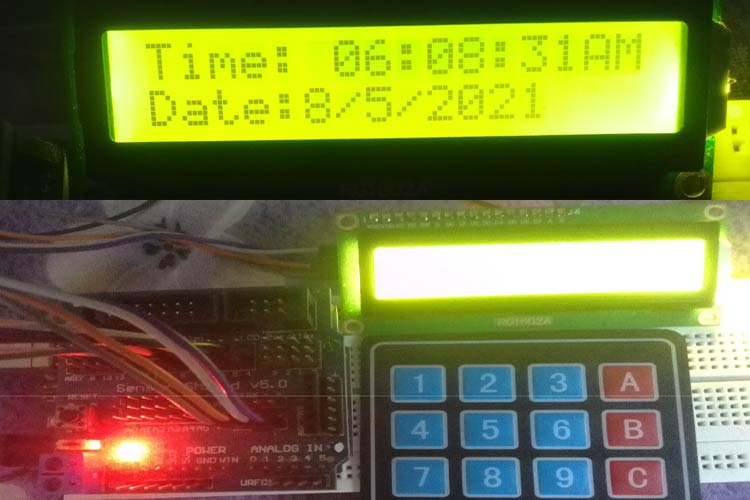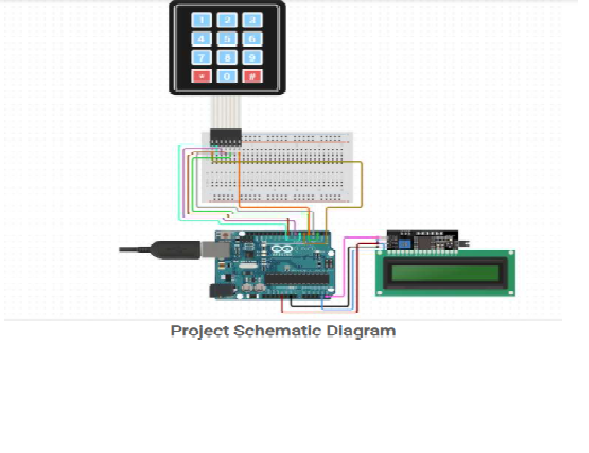797 2
Soumyajoy Debnath

## Digital Clock using Arduino UNO Without RTC Module

In this project we have the above mentioned components and the working is quite east to understand...

Arduino IDE

### Project Hardware Software Selection``` ```
```#include <Wire.h> #include <LiquidCrystal_I2C.h> #include<Keypad.h> int clock(int hr,int minute,int sec); int date(int hr,int minute,int sec); int i,j,k,hr1,minute1,sec1; int flag=0; // Set the LCD address to 0x27 for a 16 chars and 2 line display LiquidCrystal_I2C lcd(0x3F, 16, 2); const byte ROWS = 4; //four rows const byte COLS = 4; //three columns char keys[ROWS][COLS] = {   {'1','2','3','A'},   {'4','5','6','B'},   {'7','8','9','C'},   {'*','0','#','D'} }; byte rowPins[ROWS] = {11, 10, 9, 8}; //connect to the row pinouts of the keypad byte colPins[COLS] = {7, 6, 5, 4}; //connect to the column pinouts of the keypad```
``` Keypad keypad = Keypad( makeKeymap(keys), rowPins, colPins, ROWS, COLS ); void setup() {     lcd.begin();     lcd.backlight();     Serial.begin(9600);   lcd.setCursor(0,0);   lcd.print("WELCOME,");   lcd.setCursor(0,1);   lcd.print("To Digital Clock");  delay(2000);   lcd.clear();   delay(1100);   } void loop(){    if(flag==0){    hr1=sethour();    minute1=setmin();    sec1=00;}    }    clock(hr1,minute1,sec1);   }   int sethour()                  //for setting the hour format   {     lcd.setCursor(0,0);     lcd.print("Press # To");     lcd.setCursor(0,1);     lcd.print("Set HOUR:");     int val1= getKeypadIntegerMulti();     Serial.println("Value is");     Serial.println(val1);          delay(500);          lcd.clear();     delay(500);     return val1;        }    int setmin()                //for setting the minute format   {     lcd.setCursor(0,0);     lcd.print("Press # To");     lcd.setCursor(0,1);     lcd.print("Set MINUTE:");     int val2= getKeypadIntegerMulti();          Serial.println("Value is");     Serial.println(val2);          delay(500);          lcd.clear();     delay(500);     return val2;    }   long getKeypadIntegerMulti() {     long value = 0;                                // the number accumulator   long keyvalue;                                     // the key pressed at current moment   int isnum;   Serial.println("Enter the digits ");   Serial.print("You have typed: ");     do   {     keyvalue = keypad.getKey();                          // input the key     isnum = (keyvalue >= '0' && keyvalue <= '9');         // is it a digit?     if (isnum)     {       Serial.print(keyvalue - '0');       lcd.print(keyvalue - '0');       value = value * 10 + keyvalue - '0';               // accumulate the input number     }   } while (isnum || !keyvalue);                          // until not a digit or while no key pressed   //     Serial.println(" ");   Serial.print("Returning from funtion: ");   Serial.println(value);   return value; }//getKeypadInteger     int clock(int hr,int minute,int sec)  {          if(hr==0  || minute<60 && sec==0 || sec<60)        {         // i loop for  day hour    for(i=hr;i<24;i++)     {           // j loop for day mins   for(j=minute;j<60;j++)  {                                            //k loop for day seconds         for(k=sec;k<60;k++)      {            lcd.print("Time: ");            if(i<=9){    // for hour setting if 0  to 9 then set 0 in front of them like 01,02,03 etc.        lcd.print("0");          }      lcd.print(i,DEC);      lcd.print(":");                                                                                        if(j<=9){             lcd.print("0");          }      lcd.print(j,DEC);      lcd.print(":");                                                              if(i<12)                    {       if(k<=9)       {         lcd.print("0");                           }                                                                                         lcd.print(k);      lcd.print("AM");     }                                             else                 {       if(k<=9)       {         lcd.print("0");                           }                                                                                         lcd.print(k);      lcd.print("PM");     }                                                                                           delay(100);             lcd.clear();                 }        sec=0;        minute=0;                         lcd.clear();     }            }        }    lcd.clear();     hr=0;     minute=0;     sec=0;        flag=1;     }         ```